Science Research
Volume 3, Issue 4, August 2015, Pages: 150-169

Optimisation of Reflow Profile of Surface Mount Assembly Using Taguchi Design of Experiments

1Department of Mechanical Engineering, Kwame Nkrumah University of Science & Technology, Kumasi, Ghana

2Electronics Manufacturing Engineering Research Group, School of Engineering, University of Greenwich, Chatham Maritime, Kent, UK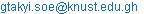(G. Takyi)

Gabriel Takyi, Peter Kojo Bernasko. Optimisation of Reflow Profile of Surface Mount Assembly Using Taguchi Design of Experiments. Science Research. Vol. 3, No. 4, 2015, pp. 150-169. doi: 10.11648/j.sr.20150304.13

Abstract: A major source of concern in the electronic manufacturing industry is the reliability of solder joints produced by lead-free solders pastes. Research has shown that solder joint produced with a thin layer of intermetallic compounds (IMC) during the reflow process has a higher reliability. The focus of this paper is on the development of a deeper understanding of the lead-free reflow soldering process and to optimise the reflow profile using Taguchi Design of Experiments (DOE). The optimum parameters are those that yield lower IMC layers. The experiment was designed to consider the effect of the following reflow soldering parameters: soak temperature, time above liquidus, soak time and time to peak temperature. The eight (8) reflow profiles studied were derived from the Taguchi DOE which also included three factorial interactions. A stencil with three (3) holes of diameters 3.1 mm, 3.7 mm and 4.3 mm was used in the solder printing process, after which the samples were reflowed. The samples were then thermal cycled, cross-sectioned and finally the IMC image captured and analysed using an optical electron microscope. The analysis of variance (ANOVA) carried out on the results indicate the soak time to be the most significant factor in achieving a solder joint with a thin IMC layer. The next most significant factor was the time above liquidus, and the most significant interaction was found to be the interaction between the soak temperature and time above liquidus. The results indicate a soak time of 60 seconds and time above liquidus of 60 seconds is preferred to achieve a thin IMC layer. The recommended time to peak temperature is 240 seconds and the soak temperature is 150°C. The results can be very useful to researchers and scientists in the surface mount technology (SMT) field, providing an understanding of the effect of the four reflow parameters studied. In addition, the DOE methodology used in this research can be adopted by other process improvement engineers or scientists involved in the optimisation of a process or product.

Keywords: Design of Experiment, Interaction Effects, Solder Volume, Reflow Soldering, Lead-free Solder

Contents

1. Introduction

One of the most common failures in electronic components is attributed to the failure of solder joints due to fatigue. Studies have shown that the characteristics lifetime of solder joint depends on the evolution of the microstructure.

Optimization and control of the reflow profile is the key to achieving good solder joints. One of the critical issues to be addressed in the transition from SnPb pastes to lead-free paste is the effect of reflow profile on the joint solderability. This is because the reflow profile significantly influences the wetting and microstructure of the solder joint. Solder pastes require an adequate reflow temperature to melt, wet, and interact with the copper pad or other board metallization and component metallization to form the solder joint. Hence, a good reflow profile is therefore essential to form a good solder joint . The reflow profile significantly affects the reliability of a solder joint because it is one of the factors that affect the formation of IMC in the solder joint . The IMC layer indicates that a good metallurgical bond has been formed between the molten solder and substrate surface. The IMC has the disadvantage of generally being the most brittle part of the solder joint . A thick IMC layer will weaken the bond, making it too brittle. Due to this, there is a great deal of interest in the effect of IMC on joint reliability . Cu/Al IMC effect on bondability using a correlation of shear strength and IMC thickness was investigated . The effect of pad sizes on IMC formation and growth of Ag/Cu solder on Cu metallization was also investigated . The IMC thickness was found to increase with ageing time.  In the study reported in reference , observations were made showing that with the increasing use of lead-free solders, reflow profiling becomes more demanding. The higher melting points (Tm) of 217°C and the smaller process windows than those of leaded solders contribute to the challenges. The research carried out and reported in  is closely related to work reported in this project.

However, how to adjust the process variables of each heating zone during reflow to ensure the proper reflow profile for all of the solder joints is a significant challenge. The main effect plots were used to identify significant parameters that were not detected by normal probability plots in the evaluation of the effect of reflow profile parameters on solder joint shear strength . The introduction of lead-free solder pastes makes the problem more complicated, due to a higher reflow temperature and therefore narrower process window than that of SnPb solder pastes .

Reflow profiling for lead free solder alloys has been extensively studied, for example in references , , and . Reference  focuses on the study of the reflow profile of SnAgCu lead free solder alloy and concluded that the main factor that affects the reflow profile of SnAgCu is the peak temperature (230°C). An extensive study on reflow soldering processes and troubleshooting was carried out in . However, there have been very limited studies on the optimization of the reflow profile for lead-free soldering using Taguchi’s design of experiments. The goal of this work is to determine the reflow parameters that are most significant and results in a solder joint with thin IMC layer. Poor design of reflow parameters, for instance low temperature, will result in a poor solder joint due to poor mating of solder bumps .

The defect mechanism analysis is one of the methods that can be used to optimise a reflow profile . This reflow optimisation method requires that the shape of the temperature profile of a solder joint that is to be reflowed should match that of the target profile to avoid any defects during soldering. Three predictive methods can be used to facilitate the optimisation and control of the shape of a reflow profile to match a target profile. The methods are: Simulation methods, Statistical process control (SPC) methods and Artificial intelligence (AI) based methods . This project is concerned with the use of Statistical Process Control (SPC) method, specifically Taguchi Design of Experiment (DOE) to optimise the reflow profile. The Taguchi method of off-line quality control encompasses all stages of product /process development. However, the key element for achieving high quality at low cost is Design of Experiments (DOE). Quality achieved by means of process optimization is found by many manufacturers to be cost effective in gaining and maintaining a competitive position in the world market .

In order to achieve a good solder joint, the following pointers serve as a guide during the actual iterative reflow process: A slow ramp up rate is required to reduce hot slump, bridging, tombstoning, skewing, wicking, opens, solder beading, solder balling and component cracking. A minimized soaking zone reduces voiding, poor wetting, solder balling and opens. Use of low peak temperature reduces charring, delamination, intermetallics, leachin, dewetting and grain size.

The optimized profile requires the temperature to ramp up slowly until reaching about 180ºC. It is then gradually raised further to186ºC within about 30 seconds, then rapidly until reaching about 220ºC. At this point the temperature is brought down at a rapid cooling rate. Implementation of the optimized profile requires the support of a heating efficient reflow technology with a controllable heating rate. The convection reflow provides a controllable heating rate, is not sensitive to variation in components thus allowing realization of the optimised profile .

Full and Fractional Factorial DOE

Full factorial is the idea of designing experiments to cover all possible conditions. A design where all possible combinations of factor levels are present is called full factorial design . If all possible combinations of factor levels were tested, the average behaviour determined would be more reliable. However, conducting full factorial experiments is often costly and prohibitive. If there are k factors each at 2 levels, then the number of runs will be 2k runs. As the number of factors in a 2k factorial design increases, the number of runs required for a complete replicate of design rapidly outgrows the resources of most experimenter. If the experimenter can reasonably assume that certain higher order interactions are negligible, information on the main effects and low-order interactions may be obtained by running a fraction of the complete factorial experiment. The fractional factorial designs are one of the most widely used type of designs for products and process design and for process improvement. The successful use of fractional design is based on three key ideas: the sparsity-of-effects principle (a system is usually dominated by main effects and low-order interactions), the projection property and the sequential experimentation .

Consider a situation where seven factors, each at two levels is of interest, but the experimenter cannot afford to run all the 27 = 128 test conditions. However, 64 runs can be afforded. This suggests a one half fraction of a 27 design. Hence, the fractional design would yield 27-1= 64 test conditions. The use of the fractional factorial design reduces the required number of experiments by half. The fractional factorial design is commonly referred to as a 2k-1design.

2. Experimental Procedure

A number of experimental runs have been conducted to find out the effect of various process parameters on reflow soldering using the Qualitek-4 (QT-4) software. Based on these tests and from information from previous research studies, four key process parameters and their corresponding low and high levels have been selected. Table 1.0 shows the process parameters and their corresponding low and high levels. It is important to note that throughout the experiment the low and high levels are denoted by (1) and (2) respectively.

In this study, a Heraeus HC4020 Thermal Cycling Chamber was used to isothermally age the test samples. The machine has the capability to adjust temperatures within its chambers from -40°C to 180°C. The metallographic preparation involves 4 steps which are: cutting, mounting, grinding, and polishing. The Reichert Electron Microscope was used for observing and analysing the microstructure of the solder joints. The magnification power used was 500. The microscope has a computer and video recorder attached to it with a pre-installed visual software package ‘Image-Pro Express’ which is used in measuring the thickness of the IMC layer which is used as the response variable in this study. The video recorder transmits the live image to the PC screen. The video images of the cross sectioned materials are not reported in this study.

Table 1. Process Parameters.

 Process Parameters (Controllable Factors) Levels Low (1) High (2) a = Soak temperature 150°C 180°C b = Time above Liquidus 60 sec. 120 sec. c = Soak Time 60 sec. 120 sec. d = Time to peak Temperature 240 sec. 360 sec.

However, other important parameters considered during the experiment are: cooling rate and peak temperature as shown in Table 2.0. The cooling rate and peak temperature are maintained at 4°C/sec and 230°C throughout the duration of the experiment.

Table 2. Other Important Parameters.

 Factors kept Constant Low (1) High (2) Avg. Cooling Rate (°C/sec) 2 6 4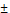2 Peak Temperature (°C) 220 240 23010

The array selected is the smallest to do the job. There are 4 two level factors and 3 interactions selected for this study as shown in Table 3.0.

Table 3. Factors and Interactions.

 s/n Factors (with 2 levels) Interactions 1 A: Soak Temp (150°C, 180°C) A: Soak Temp × B: TAL 2 B: Time above Liquidus TAL (60s, 120s) B: TAL × C: Soak Time 3 C: Soak Time (60s, 120s) A: Soak Temperature × C: Soak Time 4 D: Time to Peak Temp (240s, 360s)

Hence, an array with seven columns or more is desired. L-8 is the smallest among several arrays that meet the requirement. Thus, the L-8 array is selected. It may be noted by selecting the L-8 array, the maximum number of experimental conditions is already known as it equals the number of rows (the number associated with the array designation, L). Table 4.0 shows the L-8 orthogonal array with 4 two level factors and 3 interactions assigned. Table 5.0 shows the detailed experimental layout.

Table 4. L-8 (27) Orthogonal Array with 4 two level factors and 3 interactions assigned.

 Experiment Column A B A × B C A × C B × C D 1 1 1 1 1 1 1 1 2 1 1 1 2 2 2 2 3 1 2 2 1 1 2 2 4 1 2 2 2 2 1 1 5 2 1 2 1 2 1 2 6 2 1 2 2 1 2 1 7 2 2 1 1 2 2 1 8 2 2 1 2 1 1 2

Table 5. Experimental Layout.

 Experiment No. Factors A - Soak temperature (°C) B -Time Above Liquidus (secs) C - Soak Time (secs) D - Time to Peak (°C) A B C D 1 1 1 1 1 150 60 60 240 2 1 1 2 2 150 60 120 360 3 1 2 1 2 150 120 60 240 4 1 2 2 1 150 120 120 240 5 2 1 1 2 180 60 60 360 6 2 1 2 1 180 60 120 240 7 2 2 1 1 180 120 60 240 8 2 2 2 2 180 120 120 360

SnAgCu Lead Free Solder Paste & PCB

The Type 3 Multicore SnAgCu (Tin-Silver-Copper) lead free solder paste was used in carrying out this study, as shown in Figure 1. The constituent elements of the solder paste are also shown in Figure 1.

 Paste Type Solder Paste Particle Size Metal Content Type 3 97SCLF328AGS88.5 25 - 45 µm 88.5%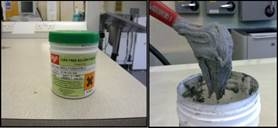Figure 1. Solder Paste Characterization.

Printed Circuit Board

Copper-clad FR4 board strips (80 mm x 120 mm) were used in this experiment. Figure 2a shows the stencil used for depositing the solder paste whilst Figure 2b shows solder paste deposited onto the copper PCB. The board has different volumes of solder ranging from the highest 4.3 mm3 to 3.1 mm3.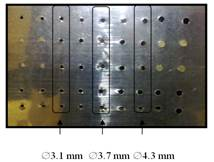Figure 2a. Electro-formed Nickel Plated Stencil.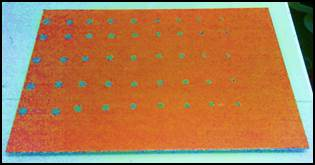Figure 2b. Cu-Clad board with solder paste deposits.

3. Results and Discussion

Optimisation of reflow profile for solder volume 1 (3.1mm3)

Volume 1 (3.1 mm3)

The eight run experimental design and results for three replicates of solder volume 1 (3 mm3) are shown in Table 6.0. The table shows the factor combinations and interactions as well as the response variable (IMC height).

Table 6. Experiment Design and Results for volume 1 (3.1 mm3).

 Experiment Factors and Interactions Results IMC Height (µm) A B A × B C A × C B × C D R1 R2 R3 1 1 1 1 1 1 1 1 2.465 1.730 2.982 2 1 1 1 2 2 2 2 3.935 3.472 3.884 3 1 2 2 1 1 2 2 3.581 4.562 5.843 4 1 2 2 2 2 1 1 4.235 4.698 3.976 5 2 1 2 1 2 1 2 2.165 4.235 3.269 6 2 1 2 2 1 2 1 5.515 2.928 4.589 7 2 2 1 1 2 2 1 2.941 2.342 2.848 8 2 2 1 2 1 1 2 6.305 2.846 4.031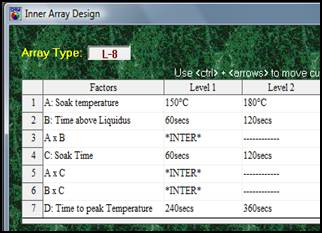Figure 3. Inner Array Design.

Figure 3 shows the details (a screen shot) of the QT-4 software of the automatically selected columns of factors and interactions. The results and S/N ratios for the conditions of Volume 1 are shown in Figure 4. The S/N ratio of trial condition 1 is calculated using the quality characteristics of smaller is better (the thinner the IMC layer thickness the better) as shown in equations 1, 2 and 3. Equation 3 shows the computation of S/N ratio for trial # 1 as shown in table 4.0. S/N ratios for trial # 2 up to 8 are computed in the same way using data from table 7.0. MSD is the mean square deviation.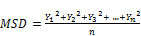(1)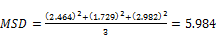(2)(3)

The S/N ratios are used to calculate the factor average effects. The average effects are obtained by dividing the total S/N ratios, at the specified level, of a particular factor by the number of results (four in this case). For example to calculate the average effect of factor A at level 1, all S/N ratios of factor A at level 1 are averaged. The average effect of Factor A is calculated as follows: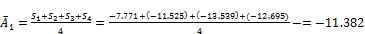(4)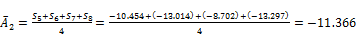(5)

Where,,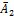are the factor levels andetc. are corresponding S/N ratio results. The average effects of other factors (B1, B2, C1, C2 etc.) are calculated in the same way and presented in Figure 5.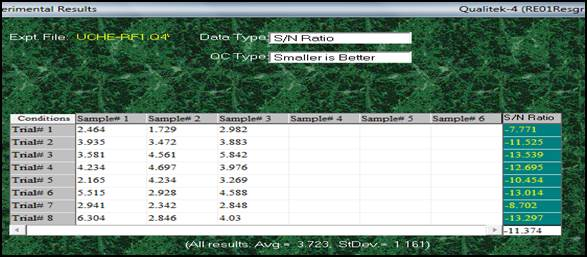Figure 4. Results and S/N Ratios.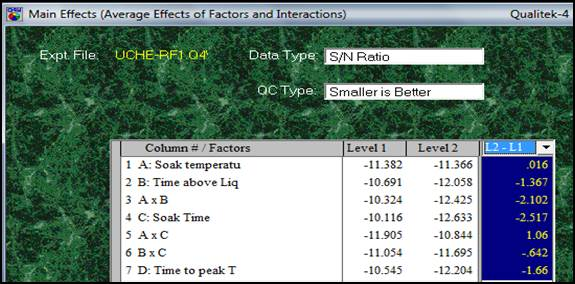Figure 5. Effects of Factors and Interactions.

Plot of Average Effects

The columns of average effects are more useful when presented in graphical format as shown in Figure 6. The average plot is obtained by plotting the average factor level effects (numerical value of results along the y axis) against the corresponding factor levels (x axis). The slope in each graph is directly proportional to the difference of the two level average effects shown in the last column of Figure 5. If the line is close to being flat (i.e. the difference is small), the effect of the factor or interaction placed in this column is smaller. The influence is absent when the line is perfectly horizontal. The slope of the plots of column effects (always a single straight line for a two-level column) also allows determination of the relative influences on each other. However, ANOVA calculations must be carried out to confirm their level of influence.

3.1. Main Effect

The main effect is describes as the trend of influence of a factor to the characteristics of measure. The values listed as main effect have only relative, not absolute, meaning. The main effect is generally expressed by the difference in the average effects at the two levels (for a two level factor) or by plotting the average effect. It is expressed numerically and computed by subtracting the first level effect from that at the second level. For example:

Main Effect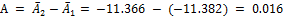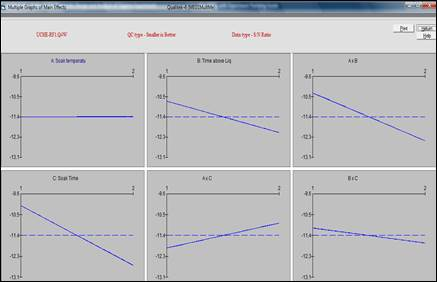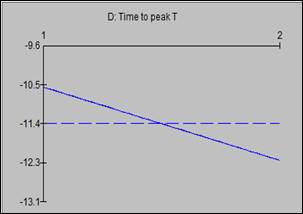Figure 6. Plot of Main Effects.

3.2. Interactions

Figure 7 shows the interaction plots of the combined effects of the pair of factors involved in the order of the strength of their presence. The most severe interaction (A: Soak temperature and B: Time above liquidus), with a severity index (SI) of 60.58%, is represented graphically in Figure 8.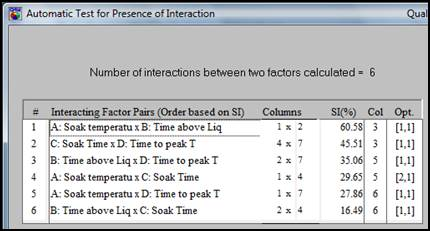Figure 7. Test for Presence of Interaction.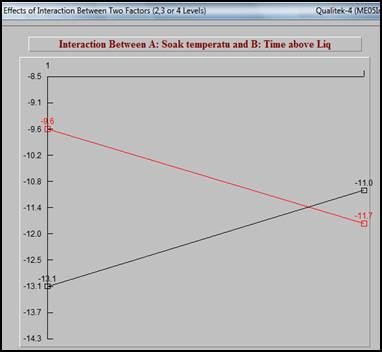Figure 8. Interaction Between factor A and factor B.

Analysis of Variance (ANOVA)

ANOVA is a statistical data treatment for sorting out the relative influence of factors to variation of results. The main objective of ANOVA is to extract from the results how much variation each factor (or interaction assigned to the column) causes relative to the total variation of the observed result.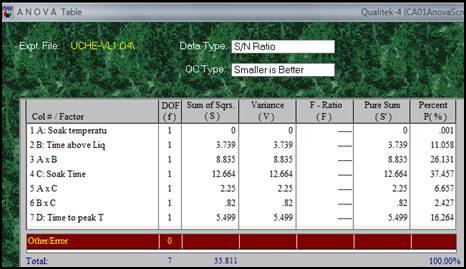Figure 9. ANOVA Results Table.

Pooled ANOVA

A pooled ANOVA table aids in identifying factors that are significant and those that are not. Pooling is a process of ignoring a factor once it is deemed insignificant. Factors that fail to pass the test of significance are regarded as insignificant and are usually treated as if they are not present. Unfortunately the test of significance can be done only when the error term has nonzero DOF. Even if a test of significance is not possible (as in this case) pooling should be considered, starting with factors with the least influence (comparing S values instead of P). A common rule is to pool a factor if its influence (S values) is less than 10% of the most influential factor. Following this rule, factor A is pooled. The pooled ANOVA table is shown in Figure 10.

The following points can be deduced from the ANOVA analysis:

1.   Factor C: soak time has the most influence (37.456%) as shown in figure 10 followed by interaction A x B and Factor D. Factor A and interaction B x C were found to be insignificant.

2.   The influence from all other external factors is 8.505%. This signifies that the influence from the pool factors, factors not included in the experiment (when these factors are not held fixed), uncontrollable factors (noise factors) and the experimental error, if any, amounts to only 8.505% of the total influence. No immediate action is required because of the magnitude of this number.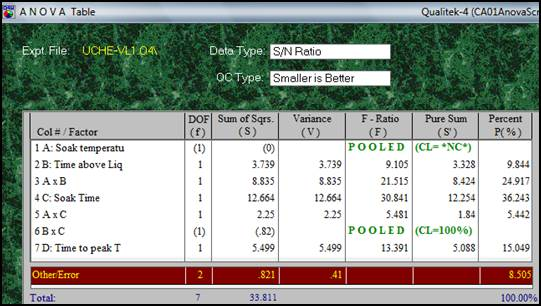Figure 10. Pooled ANOVA Table .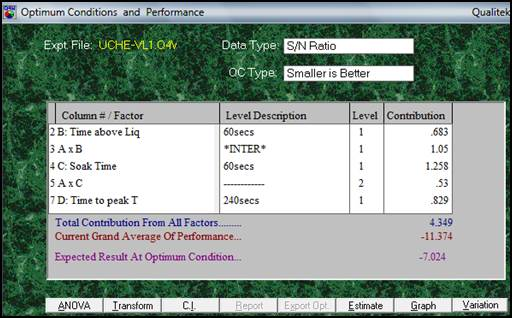Figure 11. Optimum Condition and Performance.

Optimum Condition and Performance

Figure 11 shows a plot of the optimum condition which shows two vital pieces of information: the levels of the significant factors (level 1 for factor B, C and D) and the expected performance when the factor levels are set as prescribed. The performance at optimum condition is -7.024 as shown in Figure 11. The optimum performance value indicates that if a large number of samples were tested at optimum condition, the S/N ratio of all results is expected to be close to -7.024. In S/N analysis, the expected optimum performance is always expressed in terms of S/N ratio of the performance expected at the optimum condition.

The levels of factors identified needs to be adjusted because of the interactions. Interactions found significant in this case are A x B and A x C. This adjustment can be done by reviewing interactions A x B and A x C in the interaction screen. Since factor A was found to be insignificant (shown pooled in ANOVA) it can be set at any convenient level (1 or 2) depending on which level results in an optimum condition. Level 2 for factor A was chosen for the new design as shown in Figure 12. This translates in improvement in S/N ratio (the expected result at optimum condition) from -7.024 to -6.694.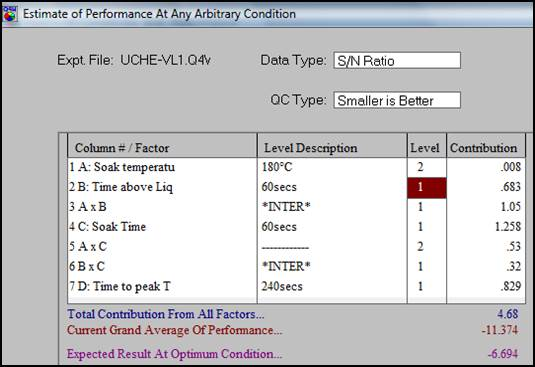Figure 12. Estimated Performance at Optimum Condition.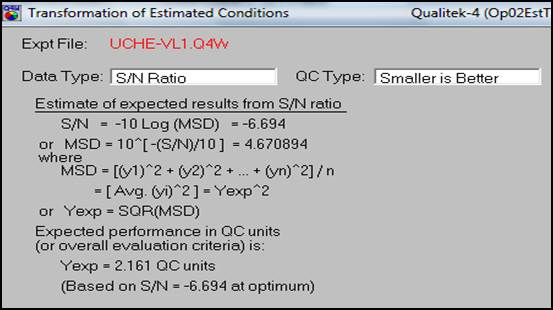Figure 13. Conversion of S/N back to original result units.

Since S/N is related to MSD and MSD is related to the results (Y), it is possible to calculate the expected result (Y) from a known S/N ratio. The QT-4 software can perform this conversion of the S/N ratio to standard values as shown in Figure 13. At the optimum estimated condition for volume 1 (3.1mm3) an IMC layer thickness of approximately 2.161µm was obtained. The final optimised reflow profile parameters of the test are shown in Table 7.

Table 7. Optimum Condition Volume 1 (3.1mm3).

 Factor Level A 2 B 1 C 1 D 1

The process capability (variation reduction) plot (Figure 14) shows the deviation from the mean of the current condition and the improved condition. The green line represents the current condition and the red line represents the improved condition.

Volume 2 (3.7 mm3)

The experimental design and analysis for volume 2 ((3.7 mm3) are exactly the same as that shown in the analysis of volume 1. Figures 15 through 24 show the results for the key steps involved. The experimental results and S/N ratio are shown in Figure 15. The S/N ratios are computed using equations 1 and 2. The S/N ratios are used to calculate the average effects of factors as indicated in the analysis of volume 1.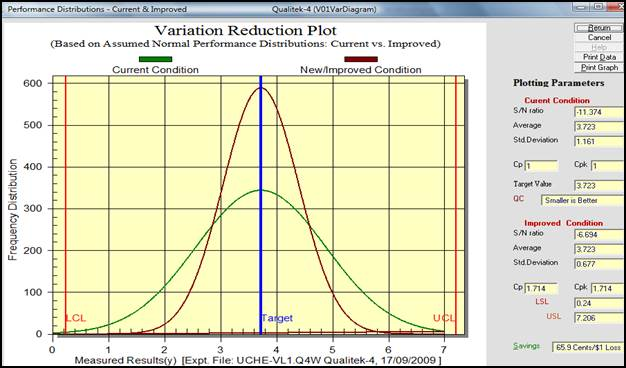Figure 14. Variation Reduction Plot (Vol 1).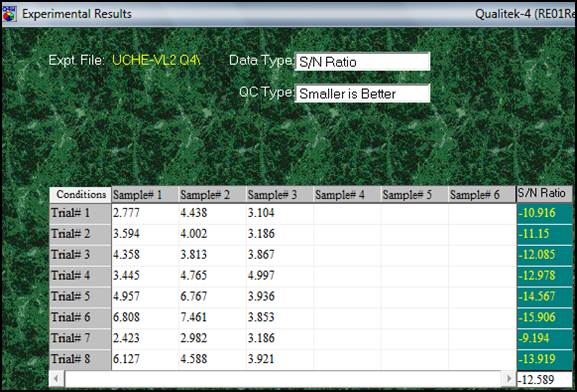Figure15. Experimental Results and S/N Ratio (Volume 2).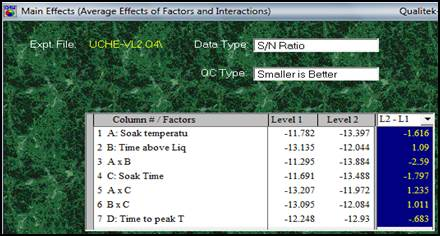Figure 16. Effects of factors and Interactions.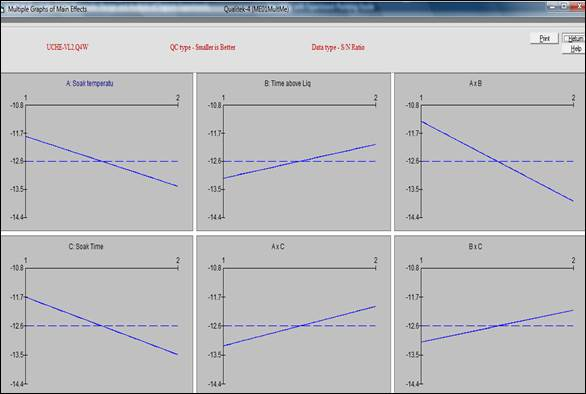Figure 17. Plot of Main Effects.

The graphical representation of column averages is shown in the main effect plot in Figure 17. The next screen shot shows the interacting factors (Figure 18).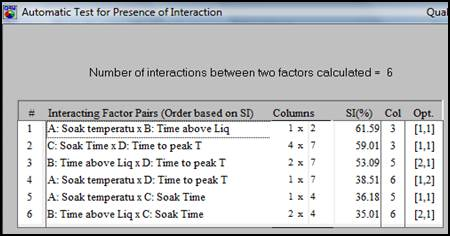Figure 18. Interaction between two Factors.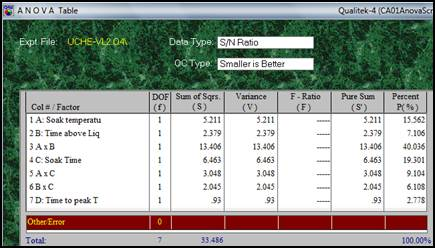Figure19. ANOVA Table.

The ANOVA table is shown in Figure 19 and the pooled ANOVA table is shown in Figure 20.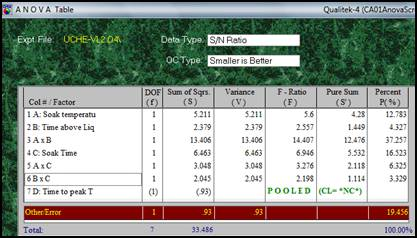Figure 20. Pooled ANOVA Table.

Figure 20 indicates that factor D (time to peak temperature) is not significant and must be dropped. The optimum condition and performance is shown in Figure 21. As shown in the analysis of volume 1, factor D is not included in the optimum condition parameters. This is so because it has very little contribution and any level set will not significantly influence the result.

The estimated performance at optimum condition is shown in Figure 22. The QT-4 automatically selects the level of factor D that results in the optimum condition. The factor level selected is 1, thereby completing the levels required to carry out an experiment.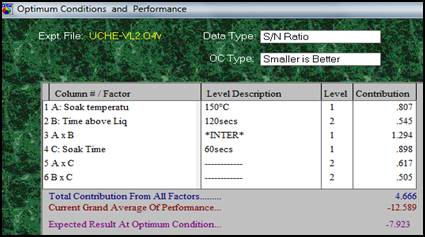Figure 21. Optimum Condition and Performance.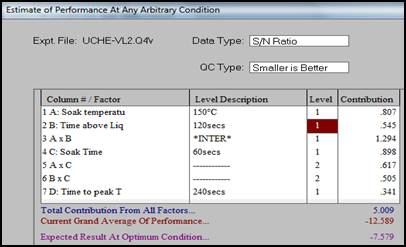Figure 22. Estimated Performance at Optimum Condition.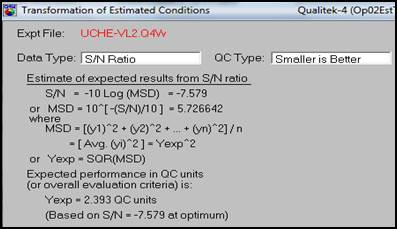Figure 23. Conversion of S/N back to original result units.

The conversion of the S/N ratio to standard values is shown Figure 23. At the optimum estimated condition for volume 2 (3.7 mm3) an IMC layer thickness of approximately 2.393 µm was obtained. The final optimised reflow profile of the test is shown in Table 8.

Table 8. Optimum Condition Volume 2 (3.7mm3).

 Factor Level A 1 B 1 C 1 D 1

As mentioned in the analysis for volume 1, the process capability (variation reduction) plot (Figure 24) shows the deviation from the mean of the current condition and the improved condition. The green line represents the current condition and the red line represents the improved condition.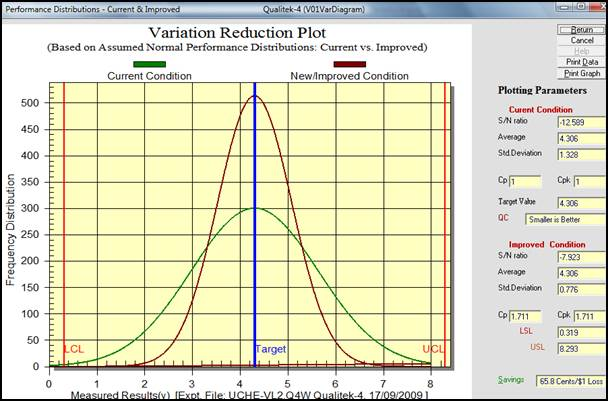Figure 24. Variation Reduction Plot.

Volume 3 (4.3mm3)

The experimental design and analysis for volume 3 is the same as that of volumes 1 and 2. The key analysis steps are described in brief below: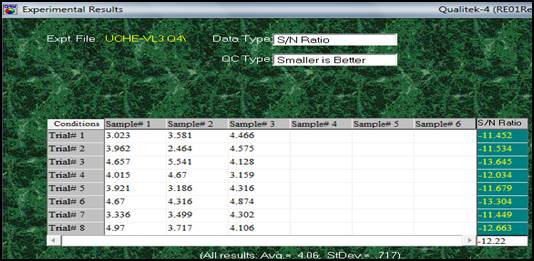Figure 25. Experimental results and S/N Ratio (Vol. 3).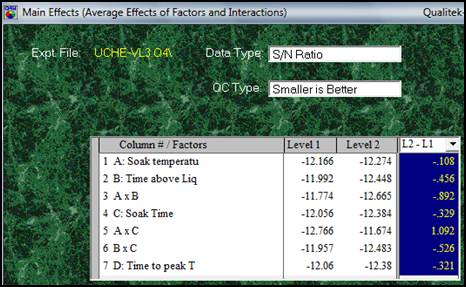Figure 26. Effects of factors and Interactions.

The experimental results and S/N ratio for volume 3 are shown in Figure 25. The effects of factors and interaction are shown in Figure 26. The graphical representation of the plot of main effects is shown in Figure 27. The interactions between factors are shown in Figure 28. It shows the severity index of each interaction effect.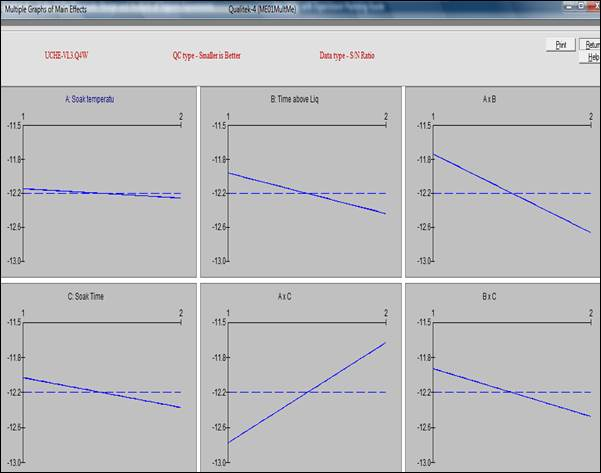Figure 27. Plot of Main Effect.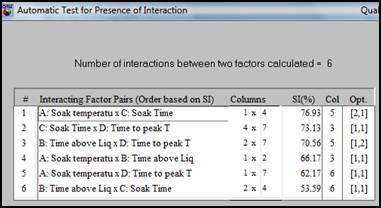Figure 28. Interaction between two Factors.

The graphical representation of the most severe factor interaction with a severity index of 76.93% is shown in Figure 29. As mentioned in the analysis of volume 1, it indicates the significance with which the interaction affects the result.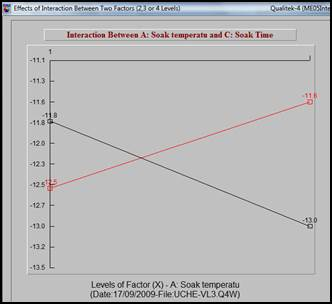Figure 29. Interaction between two Factors.

The figures 30 and 31 show the ANOVA and the pooled ANOVA table respectively. The interaction between soak time and soak temperature is found to have the highest sum of squares (2.384) followed by interaction between soak temperature and time above liquidus (1.588) as shown in figure 30. Figure 31 shows the ANOVA table indicating soak temperature as the pooled variable.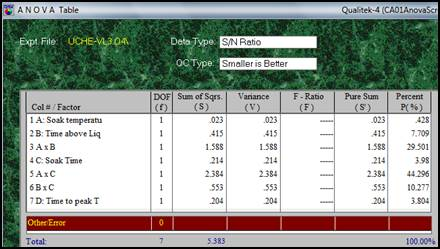Figure 30. Table of ANOVA.Figure 31. Table of Pooled ANOVA .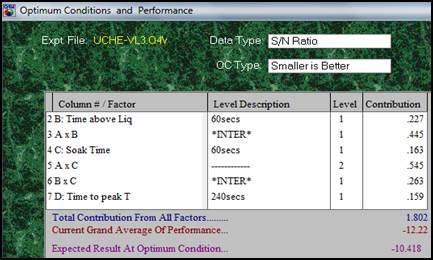Figure 32. Optimum Condition and Performance.

The optimum condition and performance is shown in Figure 32. As mentioned in the analysis of volume 1, factor A is pulled out of the table since it has little significance to the result of the experiment.

The estimated performance at optimum condition is shown in Figure 33. The QT-4 automatically selects the level of factor A that results in the optimum condition. The factor level selected is 1, thereby completing the levels required to carry out an experiment.

The conversion of the S/N ratio to standard values is shown Figure 34. At the optimum estimated condition for volume 3 (4.3 mm3) an IMC layer thickness of approximately 3.296 µm can be produced. The final optimised reflow profile of the test is shown in Table 9.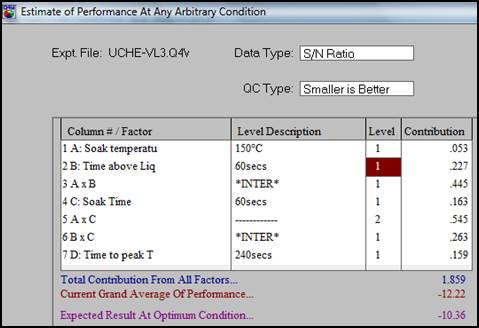Figure 33. Estimated Performance of Optimum Condition.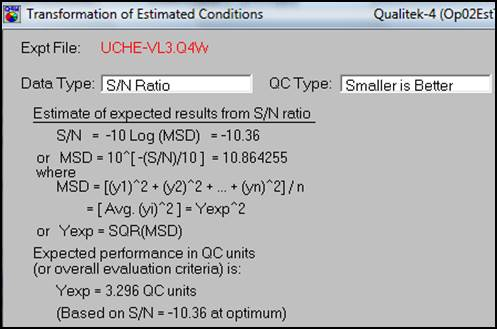Figure 34. Conversion of S/N back to original result units.

Table 9. Optimum Condition Volume 3 (4.3mm).

 Factor Level A 1 B 1 C 1 D 1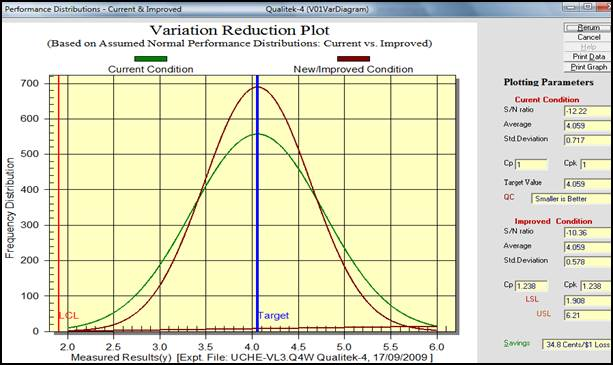Figure 35. Variation Reduction Plot.

From the process capability (variation reduction) plot shown in Figure 35, the green line represents the current condition and the red line represents the improved condition. The optimised reflow profile for the 3 respective solder joint volumes, in terms of producing a thin IMC layer thickness, are summarised in Table 10.

Table 10. Optimised Reflow Profile Parameters.

 s/n Solder Volume A: Soak Temperature (°C) B: Time above Liquidus (sec) C: Soak Time (sec) D: Time to Peak (sec) IMC Thickness 1 Volume 1 (3.1 mm3) 180 60 60 240 2.161 µm 2 Volume 2 (3.7 mm3) 150 60 60 240 2.393µm 3 Volume 3 (4.3 mm3) 150 60 60 240 3.296 µm

According to the ANOVA the most significant factor was the soak time. The results suggest a soak time of 60s and a time to peak temperature of 240 seconds for a thin IMC layer. The suggested time above liquidus (TAL) is 60 seconds and the soak temperature is 150°C.

The volume of the solder paste had very little significance on the formation of the IMC layer. It was observed that thinner IMC layers were formed with smaller volumes. The thinnest IMC layer formed during the experiment was 2.161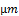. This was obtained using volume 1 (3.1 mm3) and reflow profile 1.

4. Conclusion

An Optimisation of the reflow profile of SnAgCu lead-free solder alloy has been conducted using Qualitek-4 software based on Taguchi DOE. The focus of the study was on the development of a deeper understanding of the lead-free reflow soldering process and the optimisation of the reflow profile in order to achieve a thin IMC layer of the solder joint. The most significant factor was found to be the soak time followed by time above liquidus (TAL). This was found to contrast slightly with studies carried out by Salam et al (2004) where the peak temperature and cooling rate were found to be the most significant factors. The difference could be attributed to the different experimental design set up. In this experiment, the peak temperature and cooling rate were not among the process parameters considered. The results suggest a soak time of 60 seconds and time above liquidus of 60 seconds. The recommended time to peak temperature is 240 seconds and the soak temperature is 150°C.

The results show that a shorter TAL of 60 seconds is preferred. In previous studies, shorter time above liquidus of 40 seconds was recommended (Salam et al 2004). This is because the longer the time spent above the melting point (217°C) of the SnAgCu solder paste, the larger the IMC layer produced. The most significant interaction during the experiment was the soak temperature and the TAL. At the optimum estimated condition for volume 1 (3.1 mm3) an IMC layer thickness of approximately 2.161µm was obtained. In volume 1, this interaction (soak temperature and TAL) was the second most significant contributor. The soak time, was the largest contributor. The optimum estimated condition for volume 2 (3.7 mm3) yielded an IMC layer thickness of approximately 2.393µm. Here the interaction between soak temperature and TAL was the largest contributor to the formation of a thin IMC. In volume 3 (4.3 mm3), an IMC thickness of 3.296was obtained at the optimum condition of reflow profile. The interaction between the soak temperature and soak time was the most significant contributor, followed by the interaction between soak temperature and TAL. The results generally indicate that the larger the volume, the higher the IMC thickness. There has been very limited research carried out on the effect of factor interactions as it relates to IMC formation and further study is thus recommended in this area. The study of the effect of interaction on the formation of IMC layer is the novelty of this project.

Acknowledgement

The authors would like to acknowledge the support provided by staff and researchers of EMERG, University of Greenwich for the work reported in this paper.

References

1. J. Pan, B. Toleno, T. Chou, and W. Dee (2006) "The effect of reflow profile on SnPb and SnAgCu solder joint shear strength", Soldering & Surface Mount Technology, Vol. 18 No. 4, pp. 48 -56
2. B. Salam, C. Vireseda, H. Da, N.N Ekere, (2004) "Reflow profile study of the Sn-Ag-Cu solder", Soldering and Surface Mount Technology, Vol. 16 No. 1, pp. 27 – 34.
3. Salam, B., Ekere, N.N., Rajkumar, D. (2001) "Study of the interface microstructure of SnAgCu lead free solder and the effect of solder volume on intermetallic layer formation", Proceedings of the Electronics Components and Technology Conference, IEEE 16 No. 1.
4. A. Haseeb, T.S Leng, (2011), Effects of Co nanoparticle addition to Sn-3.8Ag-0.7Cu solder on interfacial structure after reflow and ageing, Intermetallics, vol. 19, iss. 5, pp, 707–712, Elsevier.
5. S. Na, T. Hwang, J. Park, J.Y Kim, (2011), "Characterization of IMC growth in Cu wire ball bonding on Al pad metallization", 61stIEEE Electronic components and technology conference, ECTC, Lake Buena Vista FL. pp. 1740-1745.
6. P. Bernasko, M. Sabuj, N. Ekere, G. Takyi, (2011), "Evaluating the effect of pad sizes on the inter-metallic layer formation and growth for Sn-Cu-Ag solders on Cu metallization", international conference on electronics packaging (ICEP), Japan,
7. G. Takyi, P.K Bernasko, (2014), The Effects of reflow profile parameters on Sn-Ag-Cu solder bumps and Cu substrate using full factorial design, Journal of surface mount technology, (SMT), vol. 27, iss. 4, pp 13-22
8. J. Gao, Y. Wu, H. Ding, N. Wan, (2008) "Thermal profiling: a reflow process based on the heating factor", Soldering & Surface Mount Technology, vol. 20 no. 4, pp 20 – 27.
9. D.C Whalley, (2004) "A simplified reflow soldering process model", Journals of Materials processing Technology, Vol.150, pp. 134 – 144.
10. R. Pandler, N. Jodhan, R. Raut, M. Liberatore, (2010), Electronics head in-pillow defect- role of solder ball alloy, 12thelectronics packaging technology conference.
11. N. Lee, (2001), "Reflow soldering processes and troubleshooting: SM, BGA, CSP, and flip chip technologies", Boston: Newnes, ISBN-13: 978-0-7506-7218-4
12. J. Gao, Y. Wu, H. Ding, (2007) "Optimization of the reflow profile based on the heating factor", Soldering and Surface Mount Technology, Vol. 19 No. 1, pp 28 – 33.
13. B. Yuvaraj, (2012), Application of Taguchi Method for Design of Experiment in Turning Gray Cast Iron, International Journal of Engineering Research and Applications Vol. 2, Issue 3, pp.1391-1397.
14. R.K Roy, (2001) "Design of experiments using the Taguchi approach: 16 steps to product and process improvement". New York: Wiley.
15. D. Montgomery, (2005) "Design and Analysis of Experiments", 6th Edition, Wiley: New York, ISBN:0-471-66159-7.

 Contents 1. 2. 3. 3.1. 3.2. 4.
Article ToolsAbstractPDF(1692K)